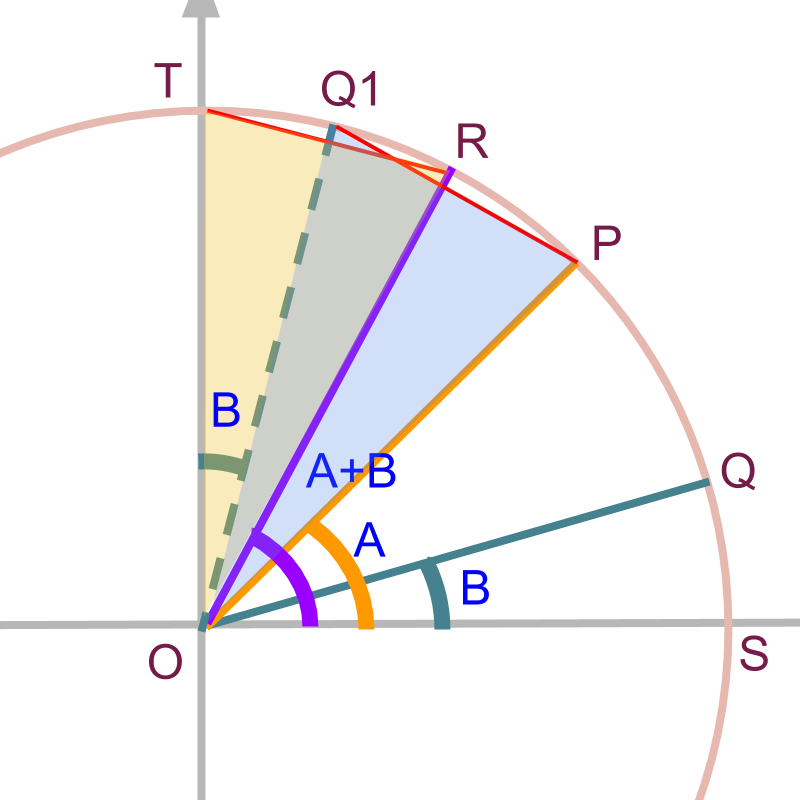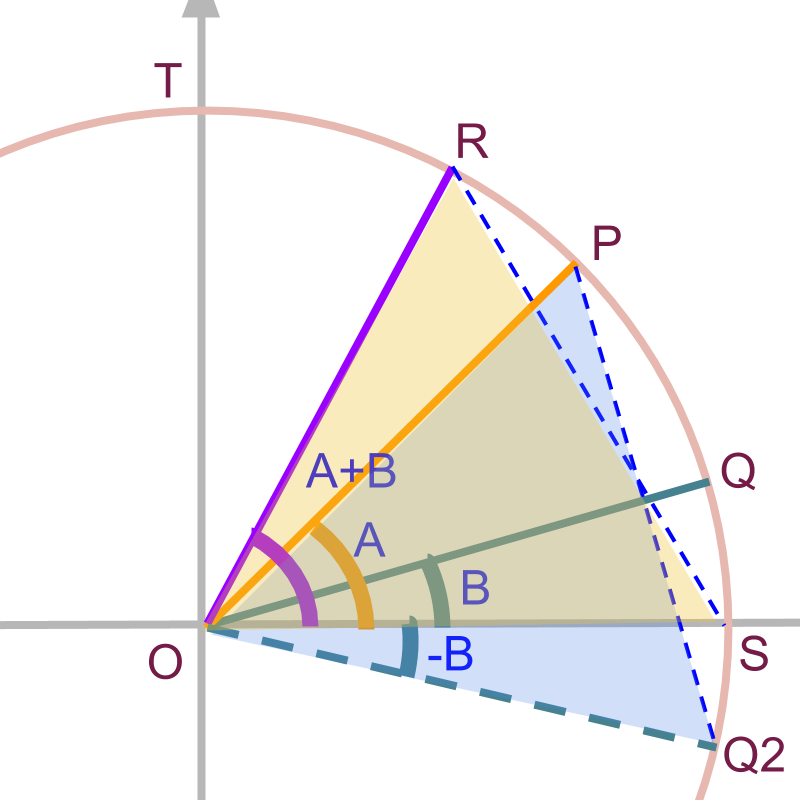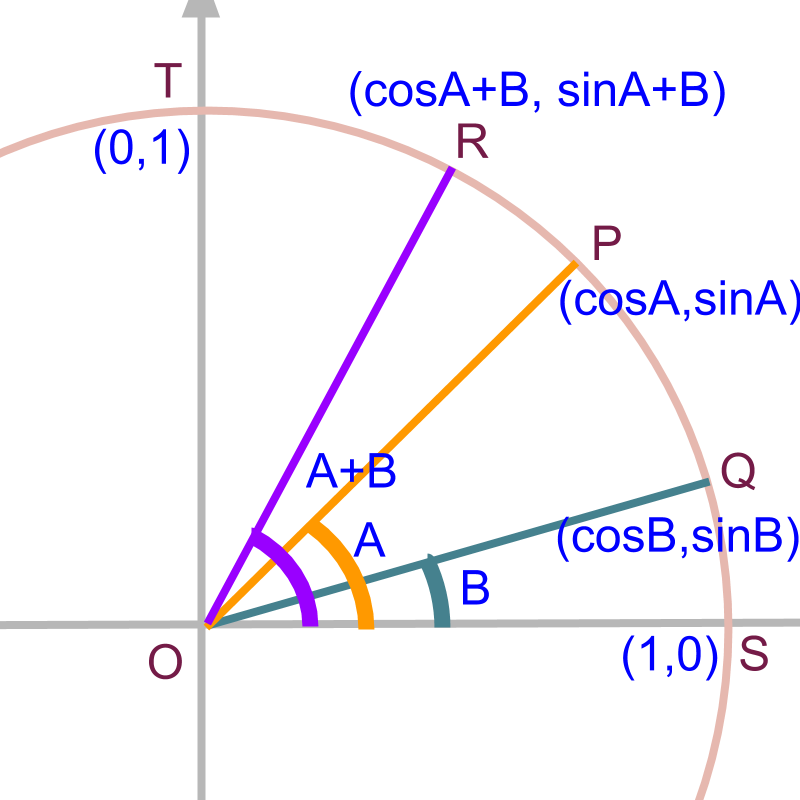Compound Angles: Geometrical Proof for $\mathrm{sin}\left(A+B\right)$$\sin \left(A + B\right)$

what you'll learn...

sin(A+B)»  the coordinates of points
→  $P\left(\mathrm{cos}A,\mathrm{sin}A\right)$$P \left(\cos A , \sin A\right)$
→  $Q\left(\mathrm{cos}B,\mathrm{sin}B\right)$$Q \left(\cos B , \sin B\right)$
→  $R\left(\mathrm{cos}\left(A+B\right),\mathrm{sin}\left(A+B\right)\right)$$R \left(\cos \left(A + B\right) , \sin \left(A + B\right)\right)$
→  $Q1\left(\mathrm{sin}B,\mathrm{cos}B\right)$$Q 1 \left(\sin B , \cos B\right)$
→  $T\left(0,1\right)$$T \left(0 , 1\right)$

»  equate the distance $\overline{RT}=\overline{PQ1}$$\overline{R T} = \overline{P Q 1}$

»  $\mathrm{sin}\left(A+B\right)$$\sin \left(A + B\right)$$=\mathrm{sin}A\mathrm{cos}B$$= \sin A \cos B$$+\mathrm{cos}A\mathrm{sin}B$$+ \cos A \sin B$

cos(A+B)»  equate the distance $\overline{RS}=\overline{PQ2}$$\overline{R S} = \overline{P Q 2}$

»  $\mathrm{cos}\left(A+B\right)$$\cos \left(A + B\right)$$=\mathrm{cos}A\mathrm{cos}B$$= \cos A \cos B$$-\mathrm{sin}A\mathrm{sin}B$$- \sin A \sin B$

problem definition

Consider points $P$$P$ and $Q$$Q$ with angles $\angle A$$\angle A$ and $\angle B$$\angle B$ in unit circle as shown in the figure.

The point $P$$P$ is $\left(\mathrm{cos}A,\mathrm{sin}A\right)$$\left(\cos A , \sin A\right)$

The point Q is $\left(\mathrm{cos}B,\mathrm{sin}B\right)$$\left(\cos B , \sin B\right)$In the given figure, point $R$$R$ is for angle $\angle \left(A+B\right)$$\angle \left(A + B\right)$.

The point R is $\left(\mathrm{cos}\left(A+B\right),\mathrm{sin}\left(A+B\right)\right)$$\left(\cos \left(A + B\right) , \sin \left(A + B\right)\right)$.

In the given figure, coordinates of $P$$P$, and $Q$$Q$ are given. Can coordinate of $R$$R$ be calculated?

In other words, given the trigonometric ratios of A and B , can we compute trigonometric ratios of $A+B$$A + B$?
$\mathrm{sin}\left(A+B\right)=?$
$\mathrm{cos}\left(A+B\right)=?$

proofIn the modified figure, point $Q1$$Q 1$ is for angle $\angle \left(90-B\right)$$\angle \left(90 - B\right)$.

The point $Q1$$Q 1$ is $\left(\mathrm{cos}\left(90-B\right),\mathrm{sin}\left(90-B\right)\right)$$\left(\cos \left(90 - B\right) , \sin \left(90 - B\right)\right)$ $=\left(\mathrm{sin}B,\mathrm{cos}B\right)$$= \left(\sin B , \cos B\right)$ As $\mathrm{cos}\left(90-B\right)=\mathrm{sin}B$$\cos \left(90 - B\right) = \sin B$ and $\mathrm{sin}\left(90-B\right)=\mathrm{cos}B$$\sin \left(90 - B\right) = \cos B$.

In the given figure, consider point $T\left(0,1\right)$$T \left(0 , 1\right)$.

the angle $\angle ROT$$\angle R O T$

$\quad \quad = \angle S O T - \angle S O R$

$\quad \quad = 90 - \left(A + B\right)$

In the given figure,

$\angle POQ1$$\angle P O Q 1$
$\quad \quad = \angle S O T - \angle S O P - \angle Q 1 O T$
$\quad \quad = 90 - A - B$

In the given figure:
$\angle ROT=90-\left(A+B\right)$$\angle R O T = 90 - \left(A + B\right)$
$\angle POQ1=90-A-B$$\angle P O Q 1 = 90 - A - B$.

That is, the angle subtended by the two chords $\overline{RT}$$\overline{R T}$ and $\overline{PQ1}$$\overline{P Q 1}$ are equal.

In the given figure, the coordinate of $R$$R$ is $\left(\mathrm{cos}\left(A+B\right),\mathrm{sin}\left(A+B\right)\right)$$\left(\cos \left(A + B\right) , \sin \left(A + B\right)\right)$ and the coordinate of $T$$T$ is $\left(0,1\right)$$\left(0 , 1\right)$. So, the Square of length of chord, using the distance formula, is

${\overline{RT}}^{2}$${\overline{R T}}^{2}$

$\quad \quad = {\left(\cos \left(A + B\right) - 0\right)}^{2} + {\left(\sin \left(A + B\right) - 1\right)}^{2}$

$\quad \quad = 2 - 2 \sin \left(A + B\right)$

Square of length of chord $\overline{RT}$$\overline{R T}$ is calculated as follows.

$T\left(0,1\right)$$T \left(0 , 1\right)$
$R\left(\mathrm{cos}\left(A+B\right),\mathrm{sin}\left(A+B\right)\right)$$R \left(\cos \left(A + B\right) , \sin \left(A + B\right)\right)$

${\overline{RT}}^{2}$${\overline{R T}}^{2}$
$\quad \quad = \textcolor{c \mathmr{and} a l}{{\left(\cos \left(A + B\right) - 0\right)}^{2}}$
$\quad \quad \quad + \textcolor{\mathrm{de} e p s k y b l u e}{{\left(\sin \left(A + B\right) - 1\right)}^{2}}$
$\quad \quad = \textcolor{c \mathmr{and} a l}{{\cos}^{2} \left(A + B\right)} + \textcolor{\mathrm{de} e p s k y b l u e}{{\sin}^{2} \left(A + B\right)}$
$\quad \quad \quad + \textcolor{\mathrm{de} e p s k y b l u e}{1 - 2 \sin \left(A + B\right)}$
$\quad \quad = 2 - 2 \sin \left(A + B\right)$

In the given figure, the coordinate of $P$$P$ is $\left(\mathrm{cos}A,\mathrm{sin}A\right)$$\left(\cos A , \sin A\right)$. and the coordinate of $Q1$$Q 1$ is $\left(\mathrm{sin}B,\mathrm{cos}B\right)$$\left(\sin B , \cos B\right)$.

The square of length of chord is

${\overline{PQ1}}^{2}$${\overline{P Q 1}}^{2}$

$\quad \quad = {\left(\cos A - \sin B\right)}^{2} + {\left(\sin A - \cos B\right)}^{2}$

$\quad \quad = 2 - 2 \cos A \sin B - 2 \sin A \cos B$

Square of length of chord $\overline{PQ1}$$\overline{P Q 1}$ is calculated as follows.

$P\left(\mathrm{cos}A,\mathrm{sin}A\right)$$P \left(\cos A , \sin A\right)$
$Q1\left(\mathrm{sin}B,\mathrm{cos}B\right)$$Q 1 \left(\sin B , \cos B\right)$

${\overline{PQ1}}^{2}$${\overline{P Q 1}}^{2}$

$\quad \quad \quad + \textcolor{\mathrm{de} e p s k y b l u e}{{\left(\sin A - \cos B\right)}^{2}}$
$\quad \quad = \textcolor{c \mathmr{and} a l}{{\cos}^{2} \left(A\right)} + \textcolor{\mathrm{de} e p s k y b l u e}{{\sin}^{2} \left(A\right)}$
$\quad \quad \quad + \textcolor{c \mathmr{and} a l}{{\sin}^{2} \left(B\right)} + \textcolor{\mathrm{de} e p s k y b l u e}{{\cos}^{2} \left(B\right)}$
$\quad \quad \quad \textcolor{c \mathmr{and} a l}{- 2 \cos A \sin B} \textcolor{\mathrm{de} e p s k y b l u e}{- 2 \sin A \cos B}$
$\quad \quad = 2 - \textcolor{c \mathmr{and} a l}{2 \cos A \sin B} - \textcolor{\mathrm{de} e p s k y b l u e}{2 \sin A \cos B}$

Equating the square of lengths of chords $\overline{RT}$$\overline{R T}$ and $\overline{PQ1}$$\overline{P Q 1}$.
$2-2\mathrm{sin}\left(A+B\right)=$$2 - 2 \sin \left(A + B\right) =$$2-2\mathrm{cos}A\mathrm{sin}B$$2 - 2 \cos A \sin B$$-2\mathrm{sin}A\mathrm{cos}B$$- 2 \sin A \cos B$

This proves $\mathrm{sin}\left(A+B\right)=\mathrm{sin}A\mathrm{cos}B+\mathrm{cos}A\mathrm{sin}B$$\sin \left(A + B\right) = \sin A \cos B + \cos A \sin B$

proof for cosineTo compute the value of $\mathrm{cos}\left(A+B\right)$$\cos \left(A + B\right)$ the enclosed figure is used and the proof is outlined below.

$P\left(\mathrm{cos}A,\mathrm{sin}A\right)$$P \left(\cos A , \sin A\right)$
$Q\left(\mathrm{cos}B,\mathrm{sin}B\right)$$Q \left(\cos B , \sin B\right)$
$R\left(\mathrm{cos}\left(A+B\right),\mathrm{sin}\left(A+B\right)\right)$$R \left(\cos \left(A + B\right) , \sin \left(A + B\right)\right)$
$S\left(1,0\right)$$S \left(1 , 0\right)$
$Q2\left(\mathrm{cos}B,-\mathrm{sin}B\right)$$Q 2 \left(\cos B , - \sin B\right)$

${\overline{PQ2}}^{2}$${\overline{P Q 2}}^{2}$
$\quad \quad = {\left(\cos A - \cos B\right)}^{2}$$+{\left(\mathrm{sin}A+\mathrm{sin}B\right)}^{2}$$+ {\left(\sin A + \sin B\right)}^{2}$
$\quad \quad = 2 - 2 \cos A \cos B + 2 \sin A \sin B$

${\overline{RS}}^{2}$${\overline{R S}}^{2}$
$\quad \quad =$${\left(\mathrm{cos}\left(A+B\right)-1\right)}^{2}$${\left(\cos \left(A + B\right) - 1\right)}^{2}$$+{\left(\mathrm{sin}\left(A+B\right)-0\right)}^{2}$$+ {\left(\sin \left(A + B\right) - 0\right)}^{2}$
$\quad \quad = 2 - 2 \cos \left(A + B\right)$

equating these two $\mathrm{cos}\left(A+B\right)=\mathrm{cos}A\mathrm{cos}B-\mathrm{sin}A\mathrm{sin}B$$\cos \left(A + B\right) = \cos A \cos B - \sin A \sin B$

summary

$\mathrm{sin}\left(A+B\right)=\mathrm{sin}A\mathrm{cos}B+\mathrm{cos}A\mathrm{sin}B$$\sin \left(A + B\right) = \sin A \cos B + \cos A \sin B$
$\mathrm{cos}\left(A+B\right)=\mathrm{cos}A\mathrm{cos}B-\mathrm{sin}A\mathrm{sin}B$$\cos \left(A + B\right) = \cos A \cos B - \sin A \sin B$

Outline

It is advised to do the firmfunda version of "basics of Trigonometry" course before doing this.

The outline of material to learn "Advanced Trigonometry" is as follows.
Note: go to detailed outline of Advanced Trigonometry

→   Unit Circle form of Trigonmetric Values

→   Trigonometric Values in all Quadrants

→   Trigonometric Values or any Angles : First Principles

→   Understanding Trigonometric Values in First Quadrant

→   Trigonometric Values in First Quadrant

→   Trigonometric Values of Compound Angles: Geometrical Proof

→   Trigonometric Values of Compound Angles: Algebraic Proof

→   Trigonometric Values of Compound Angles: tan cot

→   Trigonometric Values of Compound Angles: more results